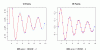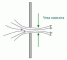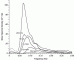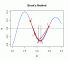I have forgotten

•http://facebook.com/
•https://www.google.com/accounts/o8/id
•https://me.yahoo.com

# Latest Content

The technical libary of numerical code and references with worked examples.

## Dispersion

engineering/fluid_mechanics/waves will
Uses a linear dispersion relationship to compute wave-frequency from wave-number
more »

## gcd

maths/combinatorics/arithmetic CodeCogs
Compute the greatest common divisor of two values. Excel: GCD
more »

## Akima

maths/approximation/interpolation lucian
Interpolates a given set of points using Akima spline fitting.more »

## Cubic

maths/approximation/interpolation CodeCogs
Interpolates a given set of points using cubic spline fitting.more »

## Introduction

engineering/fluid_mechanics/orifice CodeCogs
Introduction to orificemore »

## Pierson Moskowitz

engineering/fluid_mechanics/waves/spectra lloyd
Defines the Pierson Moskowitz spectra in the wave-frequency domainmore »

## fresnel sin

maths/special will
Fresnel sin integral.
more »

## Brent

maths/optimization lucian
Calculates the minimum of a one-dimensional real function using Brent's method.more »